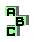SECOND BROCARD TRIANGLE

 r8 pedal triangle, centroid, similar triangles r9 circumcenter, symmedian lines r10 similar triangles r11 first Brocard triangle, centroid, perspective r21 first Brocard triangle, isotomic points, tripole d first Brocard parabola d Johnson triangle d McCay circles r679 first Brocard triangle, circummedial triangle r680 circumcircle, Brocard circle, circumcenter, Apollonian circles, inverse points r689 circumcenter, Apollonian circles, Brocard points, pedal triangle, Beltrami points, similar triangles